Anúncio

# C++_notes.pdf

24 de Mar de 2023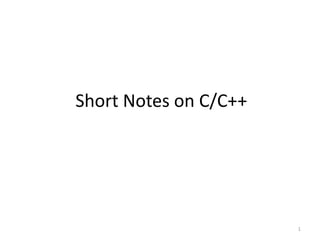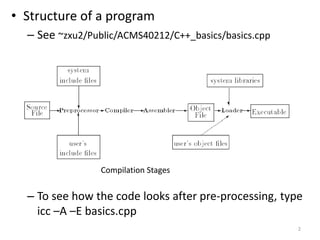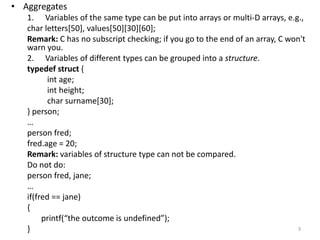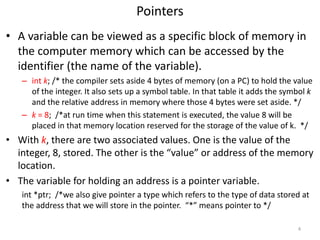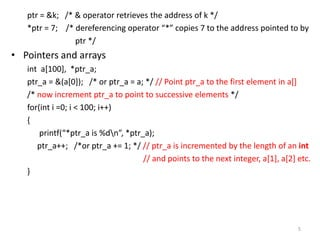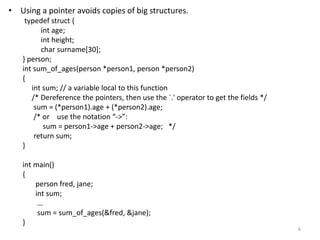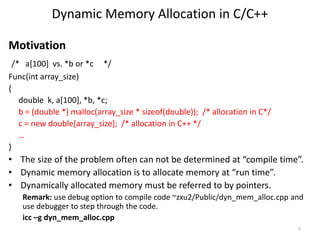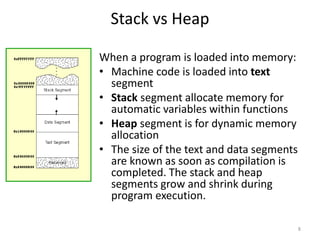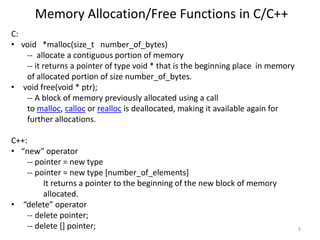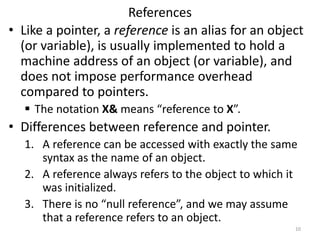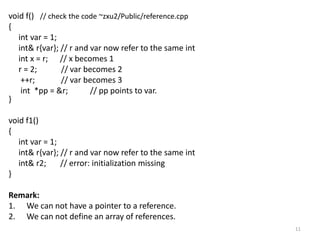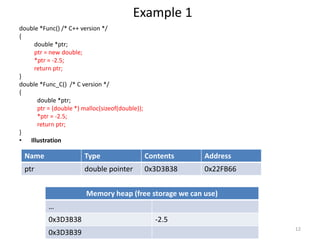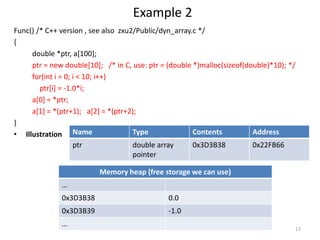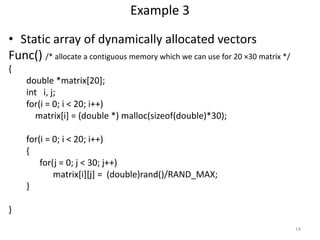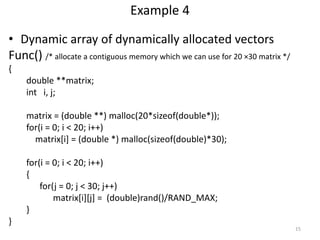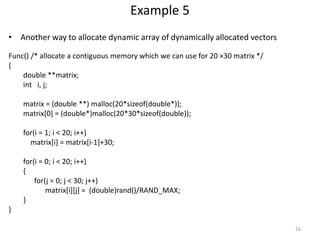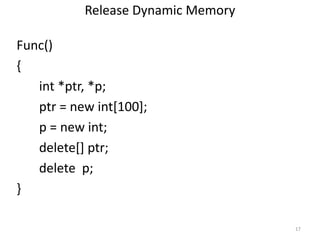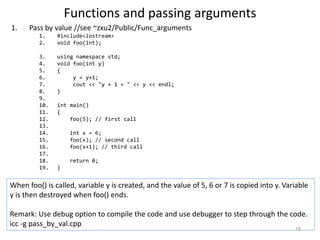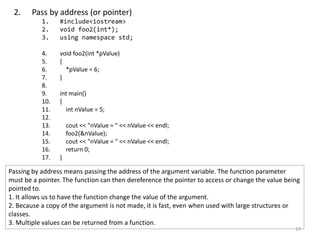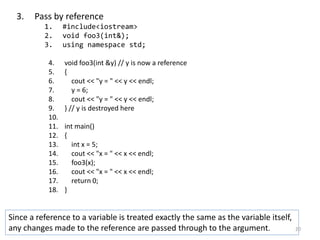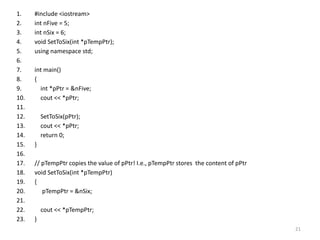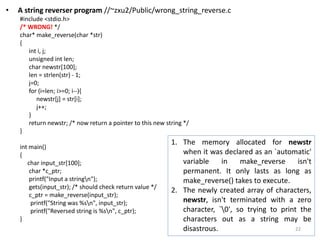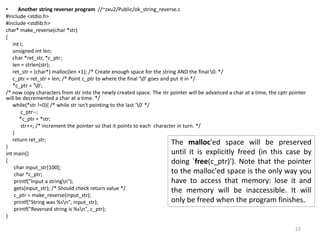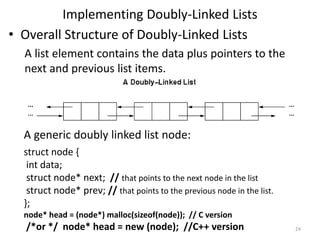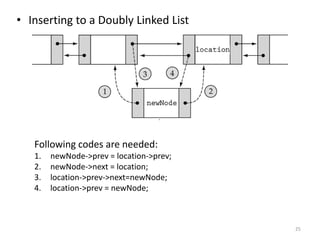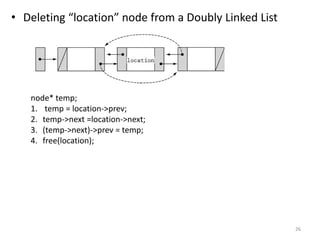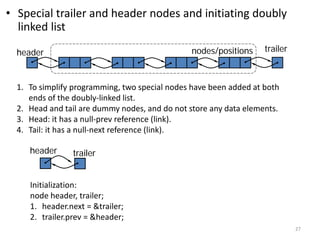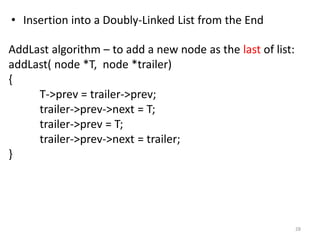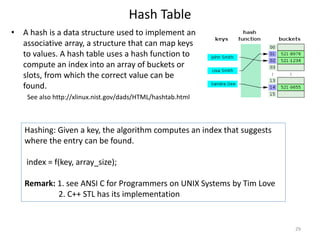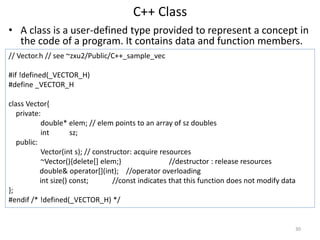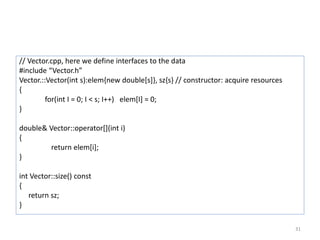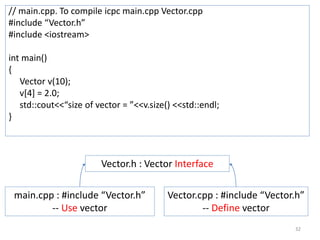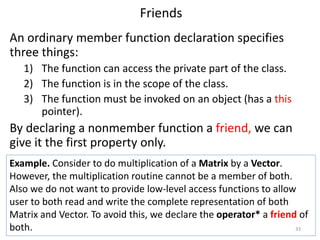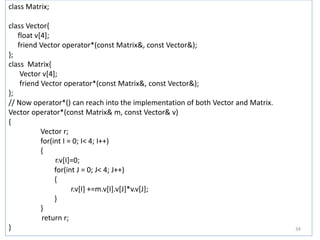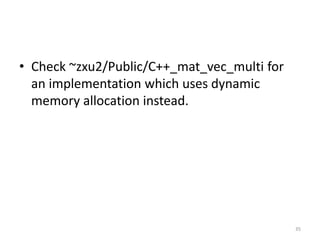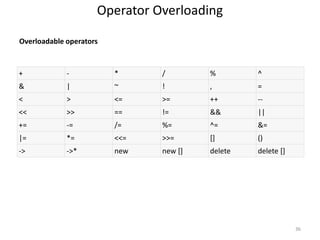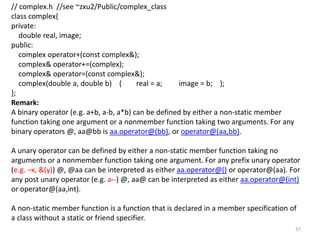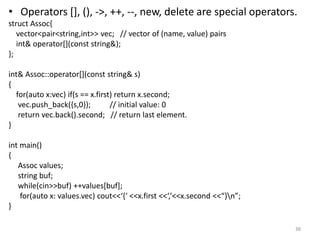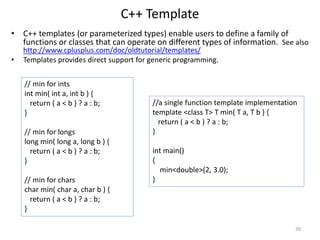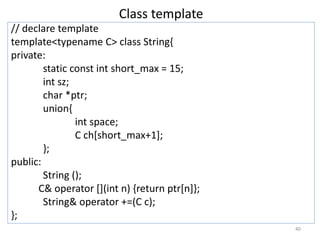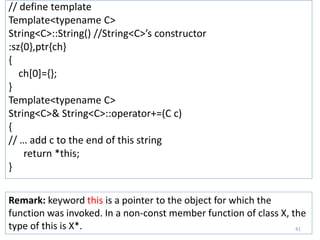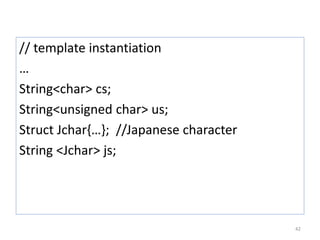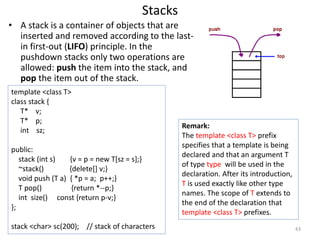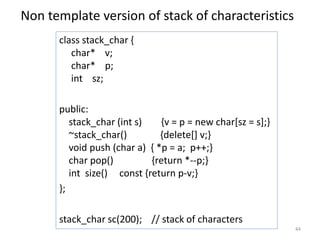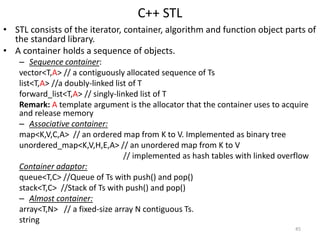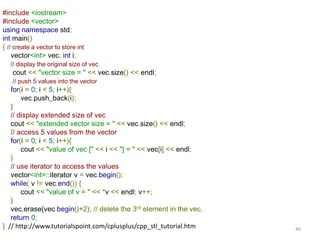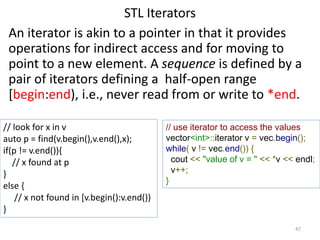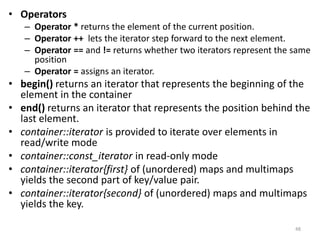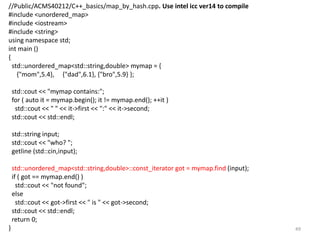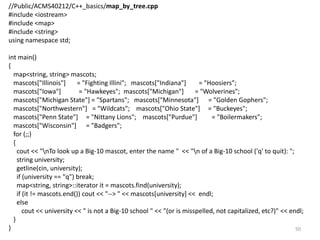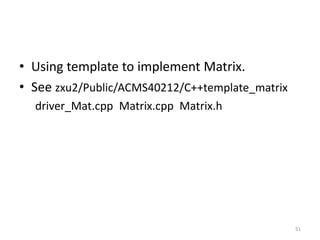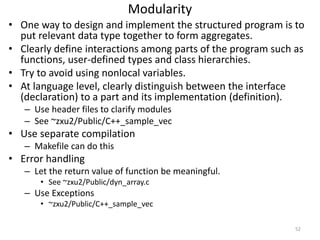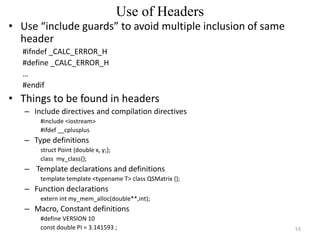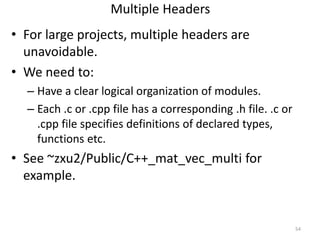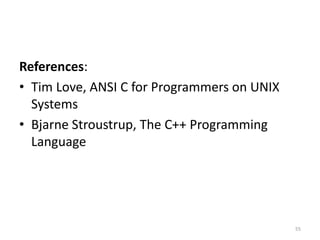1 de 55
Anúncio

### C++_notes.pdf

1. Short Notes on C/C++ 1
2. • Structure of a program – See ~zxu2/Public/ACMS40212/C++_basics/basics.cpp – To see how the code looks after pre-processing, type icc –A –E basics.cpp Compilation Stages 2
3. • Aggregates 1. Variables of the same type can be put into arrays or multi-D arrays, e.g., char letters, values; Remark: C has no subscript checking; if you go to the end of an array, C won't warn you. 2. Variables of different types can be grouped into a structure. typedef struct { int age; int height; char surname; } person; … person fred; fred.age = 20; Remark: variables of structure type can not be compared. Do not do: person fred, jane; … if(fred == jane) { printf(“the outcome is undefined”); } 3
4. Pointers • A variable can be viewed as a specific block of memory in the computer memory which can be accessed by the identifier (the name of the variable). – int k; /* the compiler sets aside 4 bytes of memory (on a PC) to hold the value of the integer. It also sets up a symbol table. In that table it adds the symbol k and the relative address in memory where those 4 bytes were set aside. */ – k = 8; /*at run time when this statement is executed, the value 8 will be placed in that memory location reserved for the storage of the value of k. */ • With k, there are two associated values. One is the value of the integer, 8, stored. The other is the “value” or address of the memory location. • The variable for holding an address is a pointer variable. int *ptr; /*we also give pointer a type which refers to the type of data stored at the address that we will store in the pointer. “*” means pointer to */ 4
5. ptr = &k; /* & operator retrieves the address of k */ *ptr = 7; /* dereferencing operator “*” copies 7 to the address pointed to by ptr */ • Pointers and arrays int a, *ptr_a; ptr_a = &(a); /* or ptr_a = a; */ // Point ptr_a to the first element in a[] /* now increment ptr_a to point to successive elements */ for(int i =0; i < 100; i++) { printf(“*ptr_a is %dn”, *ptr_a); ptr_a++; /*or ptr_a += 1; */ // ptr_a is incremented by the length of an int // and points to the next integer, a, a etc. } 5
6. • Using a pointer avoids copies of big structures. typedef struct { int age; int height; char surname; } person; int sum_of_ages(person *person1, person *person2) { int sum; // a variable local to this function /* Dereference the pointers, then use the `.' operator to get the fields */ sum = (*person1).age + (*person2).age; /* or use the notation “->”: sum = person1->age + person2->age; */ return sum; } int main() { person fred, jane; int sum; … sum = sum_of_ages(&fred, &jane); } 6
7. Dynamic Memory Allocation in C/C++ Motivation /* a vs. *b or *c */ Func(int array_size) { double k, a, *b, *c; b = (double *) malloc(array_size * sizeof(double)); /* allocation in C*/ c = new double[array_size]; /* allocation in C++ */ … } • The size of the problem often can not be determined at “compile time”. • Dynamic memory allocation is to allocate memory at “run time”. • Dynamically allocated memory must be referred to by pointers. Remark: use debug option to compile code ~zxu2/Public/dyn_mem_alloc.cpp and use debugger to step through the code. icc –g dyn_mem_alloc.cpp 7
8. Stack vs Heap When a program is loaded into memory: • Machine code is loaded into text segment • Stack segment allocate memory for automatic variables within functions • Heap segment is for dynamic memory allocation • The size of the text and data segments are known as soon as compilation is completed. The stack and heap segments grow and shrink during program execution. 8
9. Memory Allocation/Free Functions in C/C++ C: • void *malloc(size_t number_of_bytes) -- allocate a contiguous portion of memory -- it returns a pointer of type void * that is the beginning place in memory of allocated portion of size number_of_bytes. • void free(void * ptr); -- A block of memory previously allocated using a call to malloc, calloc or realloc is deallocated, making it available again for further allocations. C++: • “new” operator -- pointer = new type -- pointer = new type [number_of_elements] It returns a pointer to the beginning of the new block of memory allocated. • “delete” operator -- delete pointer; -- delete [] pointer; 9
10. References • Like a pointer, a reference is an alias for an object (or variable), is usually implemented to hold a machine address of an object (or variable), and does not impose performance overhead compared to pointers.  The notation X& means “reference to X”. • Differences between reference and pointer. 1. A reference can be accessed with exactly the same syntax as the name of an object. 2. A reference always refers to the object to which it was initialized. 3. There is no “null reference”, and we may assume that a reference refers to an object. 10
11. void f() // check the code ~zxu2/Public/reference.cpp { int var = 1; int& r{var}; // r and var now refer to the same int int x = r; // x becomes 1 r = 2; // var becomes 2 ++r; // var becomes 3 int *pp = &r; // pp points to var. } void f1() { int var = 1; int& r{var}; // r and var now refer to the same int int& r2; // error: initialization missing } Remark: 1. We can not have a pointer to a reference. 2. We can not define an array of references. 11
12. Example 1 double *Func() /* C++ version */ { double *ptr; ptr = new double; *ptr = -2.5; return ptr; } double *Func_C() /* C version */ { double *ptr; ptr = (double *) malloc(sizeof(double)); *ptr = -2.5; return ptr; } • Illustration Name Type Contents Address ptr double pointer 0x3D3B38 0x22FB66 Memory heap (free storage we can use) … 0x3D3B38 -2.5 0x3D3B39 12
13. Example 2 Func() /* C++ version , see also zxu2/Public/dyn_array.c */ { double *ptr, a; ptr = new double; /* in C, use: ptr = (double *)malloc(sizeof(double)*10); */ for(int i = 0; i < 10; i++) ptr[i] = -1.0*i; a = *ptr; a = *(ptr+1); a = *(ptr+2); } • Illustration Name Type Contents Address ptr double array pointer 0x3D3B38 0x22FB66 Memory heap (free storage we can use) … 0x3D3B38 0.0 0x3D3B39 -1.0 … 13
14. Example 3 • Static array of dynamically allocated vectors Func() /* allocate a contiguous memory which we can use for 20 ×30 matrix */ { double *matrix; int i, j; for(i = 0; i < 20; i++) matrix[i] = (double *) malloc(sizeof(double)*30); for(i = 0; i < 20; i++) { for(j = 0; j < 30; j++) matrix[i][j] = (double)rand()/RAND_MAX; } } 14
15. Example 4 • Dynamic array of dynamically allocated vectors Func() /* allocate a contiguous memory which we can use for 20 ×30 matrix */ { double **matrix; int i, j; matrix = (double **) malloc(20*sizeof(double*)); for(i = 0; i < 20; i++) matrix[i] = (double *) malloc(sizeof(double)*30); for(i = 0; i < 20; i++) { for(j = 0; j < 30; j++) matrix[i][j] = (double)rand()/RAND_MAX; } } 15
16. Example 5 • Another way to allocate dynamic array of dynamically allocated vectors Func() /* allocate a contiguous memory which we can use for 20 ×30 matrix */ { double **matrix; int i, j; matrix = (double **) malloc(20*sizeof(double*)); matrix = (double*)malloc(20*30*sizeof(double)); for(i = 1; i < 20; i++) matrix[i] = matrix[i-1]+30; for(i = 0; i < 20; i++) { for(j = 0; j < 30; j++) matrix[i][j] = (double)rand()/RAND_MAX; } } 16
17. Release Dynamic Memory Func() { int *ptr, *p; ptr = new int; p = new int; delete[] ptr; delete p; } 17
18. Functions and passing arguments 1. Pass by value //see ~zxu2/Public/Func_arguments 1. #include<iostream> 2. void foo(int); 3. using namespace std; 4. void foo(int y) 5. { 6. y = y+1; 7. cout << "y + 1 = " << y << endl; 8. } 9. 10. int main() 11. { 12. foo(5); // first call 13. 14. int x = 6; 15. foo(x); // second call 16. foo(x+1); // third call 17. 18. return 0; 19. } When foo() is called, variable y is created, and the value of 5, 6 or 7 is copied into y. Variable y is then destroyed when foo() ends. Remark: Use debug option to compile the code and use debugger to step through the code. icc -g pass_by_val.cpp 18
19. 2. Pass by address (or pointer) 1. #include<iostream> 2. void foo2(int*); 3. using namespace std; 4. void foo2(int *pValue) 5. { 6. *pValue = 6; 7. } 8. 9. int main() 10. { 11. int nValue = 5; 12. 13. cout << "nValue = " << nValue << endl; 14. foo2(&nValue); 15. cout << "nValue = " << nValue << endl; 16. return 0; 17. } Passing by address means passing the address of the argument variable. The function parameter must be a pointer. The function can then dereference the pointer to access or change the value being pointed to. 1. It allows us to have the function change the value of the argument. 2. Because a copy of the argument is not made, it is fast, even when used with large structures or classes. 3. Multiple values can be returned from a function. 19
20. 3. Pass by reference 1. #include<iostream> 2. void foo3(int&); 3. using namespace std; 4. void foo3(int &y) // y is now a reference 5. { 6. cout << "y = " << y << endl; 7. y = 6; 8. cout << "y = " << y << endl; 9. } // y is destroyed here 10. 11. int main() 12. { 13. int x = 5; 14. cout << "x = " << x << endl; 15. foo3(x); 16. cout << "x = " << x << endl; 17. return 0; 18. } Since a reference to a variable is treated exactly the same as the variable itself, any changes made to the reference are passed through to the argument. 20
21. 1. #include <iostream> 2. int nFive = 5; 3. int nSix = 6; 4. void SetToSix(int *pTempPtr); 5. using namespace std; 6. 7. int main() 8. { 9. int *pPtr = &nFive; 10. cout << *pPtr; 11. 12. SetToSix(pPtr); 13. cout << *pPtr; 14. return 0; 15. } 16. 17. // pTempPtr copies the value of pPtr! I.e., pTempPtr stores the content of pPtr 18. void SetToSix(int *pTempPtr) 19. { 20. pTempPtr = &nSix; 21. 22. cout << *pTempPtr; 23. } 21
22. • A string reverser program //~zxu2/Public/wrong_string_reverse.c #include <stdio.h> /* WRONG! */ char* make_reverse(char *str) { int i, j; unsigned int len; char newstr; len = strlen(str) - 1; j=0; for (i=len; i>=0; i--){ newstr[j] = str[i]; j++; } return newstr; /* now return a pointer to this new string */ } int main() { char input_str; char *c_ptr; printf("Input a stringn"); gets(input_str); /* should check return value */ c_ptr = make_reverse(input_str); printf("String was %sn", input_str); printf("Reversed string is %sn", c_ptr); } 1. The memory allocated for newstr when it was declared as an `automatic' variable in make_reverse isn't permanent. It only lasts as long as make_reverse() takes to execute. 2. The newly created array of characters, newstr, isn't terminated with a zero character, `0', so trying to print the characters out as a string may be disastrous. 22
23. • Another string reverser program //~zxu2/Public/ok_string_reverse.c #include <stdio.h> #include <stdlib.h> char* make_reverse(char *str) { int i; unsigned int len; char *ret_str, *c_ptr; len = strlen(str); ret_str = (char*) malloc(len +1); /* Create enough space for the string AND the final 0. */ c_ptr = ret_str + len; /* Point c_ptr to where the final '0' goes and put it in */ *c_ptr = '0'; /* now copy characters from str into the newly created space. The str pointer will be advanced a char at a time, the cptr pointer will be decremented a char at a time. */ while(*str !=0){ /* while str isn't pointing to the last '0' */ c_ptr--; *c_ptr = *str; str++; /* increment the pointer so that it points to each character in turn. */ } return ret_str; } int main() { char input_str; char *c_ptr; printf("Input a stringn"); gets(input_str); /* Should check return value */ c_ptr = make_reverse(input_str); printf("String was %sn", input_str); printf("Reversed string is %sn", c_ptr); } The malloc'ed space will be preserved until it is explicitly freed (in this case by doing `free(c_ptr)'). Note that the pointer to the malloc'ed space is the only way you have to access that memory: lose it and the memory will be inaccessible. It will only be freed when the program finishes. 23
24. Implementing Doubly-Linked Lists • Overall Structure of Doubly-Linked Lists A list element contains the data plus pointers to the next and previous list items. A generic doubly linked list node: struct node { int data; struct node* next; // that points to the next node in the list struct node* prev; // that points to the previous node in the list. }; node* head = (node*) malloc(sizeof(node)); // C version /*or */ node* head = new (node); //C++ version 24
25. • Inserting to a Doubly Linked List Following codes are needed: 1. newNode->prev = location->prev; 2. newNode->next = location; 3. location->prev->next=newNode; 4. location->prev = newNode; 25
26. • Deleting “location” node from a Doubly Linked List node* temp; 1. temp = location->prev; 2. temp->next =location->next; 3. (temp->next)->prev = temp; 4. free(location); 26
28. • Insertion into a Doubly-Linked List from the End AddLast algorithm – to add a new node as the last of list: addLast( node *T, node *trailer) { T->prev = trailer->prev; trailer->prev->next = T; trailer->prev = T; trailer->prev->next = trailer; } 28
29. Hash Table • A hash is a data structure used to implement an associative array, a structure that can map keys to values. A hash table uses a hash function to compute an index into an array of buckets or slots, from which the correct value can be found. See also http://xlinux.nist.gov/dads/HTML/hashtab.html Hashing: Given a key, the algorithm computes an index that suggests where the entry can be found. index = f(key, array_size); Remark: 1. see ANSI C for Programmers on UNIX Systems by Tim Love 2. C++ STL has its implementation 29
30. C++ Class • A class is a user-defined type provided to represent a concept in the code of a program. It contains data and function members. // Vector.h // see ~zxu2/Public/C++_sample_vec #if !defined(_VECTOR_H) #define _VECTOR_H class Vector{ private: double* elem; // elem points to an array of sz doubles int sz; public: Vector(int s); // constructor: acquire resources ~Vector(){delete[] elem;} //destructor : release resources double& operator[](int); //operator overloading int size() const; //const indicates that this function does not modify data }; #endif /* !defined(_VECTOR_H) */ 30
31. // Vector.cpp, here we define interfaces to the data #include “Vector.h” Vector.::Vector(int s):elem{new double[s]}, sz{s} // constructor: acquire resources { for(int I = 0; I < s; I++) elem[I] = 0; } double& Vector::operator[](int i) { return elem[i]; } int Vector::size() const { return sz; } 31
32. // main.cpp. To compile icpc main.cpp Vector.cpp #include “Vector.h” #include <iostream> int main() { Vector v(10); v = 2.0; std::cout<<“size of vector = ”<<v.size() <<std::endl; } Vector.h : Vector Interface main.cpp : #include “Vector.h” -- Use vector Vector.cpp : #include “Vector.h” -- Define vector 32
33. Friends An ordinary member function declaration specifies three things: 1) The function can access the private part of the class. 2) The function is in the scope of the class. 3) The function must be invoked on an object (has a this pointer). By declaring a nonmember function a friend, we can give it the first property only. Example. Consider to do multiplication of a Matrix by a Vector. However, the multiplication routine cannot be a member of both. Also we do not want to provide low-level access functions to allow user to both read and write the complete representation of both Matrix and Vector. To avoid this, we declare the operator* a friend of both. 33
34. class Matrix; class Vector{ float v; friend Vector operator*(const Matrix&, const Vector&); }; class Matrix{ Vector v; friend Vector operator*(const Matrix&, const Vector&); }; // Now operator*() can reach into the implementation of both Vector and Matrix. Vector operator*(const Matrix& m, const Vector& v) { Vector r; for(int I = 0; I< 4; I++) { r.v[I]=0; for(int J = 0; J< 4; J++) { r.v[I] +=m.v[I].v[J]*v.v[J]; } } return r; } 34
35. • Check ~zxu2/Public/C++_mat_vec_multi for an implementation which uses dynamic memory allocation instead. 35
36. Operator Overloading + - * / % ^ & | ~ ! , = < > <= >= ++ -- << >> == != && || += -= /= %= ^= &= |= *= <<= >>= [] () -> ->* new new [] delete delete [] Overloadable operators 36
37. // complex.h //see ~zxu2/Public/complex_class class complex{ private: double real, image; public: complex operator+(const complex&); complex& operator+=(complex); complex& operator=(const complex&); complex(double a, double b) { real = a; image = b; }; }; Remark: A binary operator (e.g. a+b, a-b, a*b) can be defined by either a non-static member function taking one argument or a nonmember function taking two arguments. For any binary operators @, aa@bb is aa.operator@(bb), or operator@(aa,bb). A unary operator can be defined by either a non-static member function taking no arguments or a nonmember function taking one argument. For any prefix unary operator (e.g. –x, &(y)) @, @aa can be interpreted as either aa.operator@() or operator@(aa). For any post unary operator (e.g. a--) @, aa@ can be interpreted as either aa.operator@(int) or operator@(aa,int). A non-static member function is a function that is declared in a member specification of a class without a static or friend specifier. 37
38. • Operators [], (), ->, ++, --, new, delete are special operators. struct Assoc{ vector<pair<string,int>> vec; // vector of (name, value) pairs int& operator[](const string&); }; int& Assoc::operator[](const string& s) { for(auto x:vec) if(s == x.first) return x.second; vec.push_back({s,0}); // initial value: 0 return vec.back().second; // return last element. } int main() { Assoc values; string buf; while(cin>>buf) ++values[buf]; for(auto x: values.vec) cout<<‘{‘ <<x.first <<‘,’<<x.second <<“}n”; } 38
39. C++ Template • C++ templates (or parameterized types) enable users to define a family of functions or classes that can operate on different types of information. See also http://www.cplusplus.com/doc/oldtutorial/templates/ • Templates provides direct support for generic programming. // min for ints int min( int a, int b ) { return ( a < b ) ? a : b; } // min for longs long min( long a, long b ) { return ( a < b ) ? a : b; } // min for chars char min( char a, char b ) { return ( a < b ) ? a : b; } //a single function template implementation template <class T> T min( T a, T b ) { return ( a < b ) ? a : b; } int main() { min<double>(2, 3.0); } 39
40. Class template // declare template template<typename C> class String{ private: static const int short_max = 15; int sz; char *ptr; union{ int space; C ch[short_max+1]; }; public: String (); C& operator [](int n) {return ptr[n]}; String& operator +=(C c); }; 40
41. // define template Template<typename C> String<C>::String() //String<C>’s constructor :sz{0},ptr{ch} { ch={}; } Template<typename C> String<C>& String<C>::operator+=(C c) { // … add c to the end of this string return *this; } Remark: keyword this is a pointer to the object for which the function was invoked. In a non-const member function of class X, the type of this is X*. 41
42. // template instantiation … String<char> cs; String<unsigned char> us; Struct Jchar{…}; //Japanese character String <Jchar> js; 42
43. Stacks • A stack is a container of objects that are inserted and removed according to the last- in first-out (LIFO) principle. In the pushdown stacks only two operations are allowed: push the item into the stack, and pop the item out of the stack. template <class T> class stack { T* v; T* p; int sz; public: stack (int s) {v = p = new T[sz = s];} ~stack() {delete[] v;} void push (T a) { *p = a; p++;} T pop() {return *--p;} int size() const {return p-v;} }; stack <char> sc(200); // stack of characters Remark: The template <class T> prefix specifies that a template is being declared and that an argument T of type type will be used in the declaration. After its introduction, T is used exactly like other type names. The scope of T extends to the end of the declaration that template <class T> prefixes. 43
44. Non template version of stack of characteristics class stack_char { char* v; char* p; int sz; public: stack_char (int s) {v = p = new char[sz = s];} ~stack_char() {delete[] v;} void push (char a) { *p = a; p++;} char pop() {return *--p;} int size() const {return p-v;} }; stack_char sc(200); // stack of characters 44
45. C++ STL • STL consists of the iterator, container, algorithm and function object parts of the standard library. • A container holds a sequence of objects. – Sequence container: vector<T,A> // a contiguously allocated sequence of Ts list<T,A> //a doubly-linked list of T forward_list<T,A> // singly-linked list of T Remark: A template argument is the allocator that the container uses to acquire and release memory – Associative container: map<K,V,C,A> // an ordered map from K to V. Implemented as binary tree unordered_map<K,V,H,E,A> // an unordered map from K to V // implemented as hash tables with linked overflow Container adaptor: queue<T,C> //Queue of Ts with push() and pop() stack<T,C> //Stack of Ts with push() and pop() – Almost container: array<T,N> // a fixed-size array N contiguous Ts. string 45
46. #include <iostream> #include <vector> using namespace std; int main() { // create a vector to store int vector<int> vec; int i; // display the original size of vec cout << "vector size = " << vec.size() << endl; // push 5 values into the vector for(i = 0; i < 5; i++){ vec.push_back(i); } // display extended size of vec cout << "extended vector size = " << vec.size() << endl; // access 5 values from the vector for(i = 0; i < 5; i++){ cout << "value of vec [" << i << "] = " << vec[i] << endl; } // use iterator to access the values vector<int>::iterator v = vec.begin(); while( v != vec.end()) { cout << "value of v = " << *v << endl; v++; } vec.erase(vec.begin()+2); // delete the 3rd element in the vec. return 0; } // http://www.tutorialspoint.com/cplusplus/cpp_stl_tutorial.htm 46
47. STL Iterators An iterator is akin to a pointer in that it provides operations for indirect access and for moving to point to a new element. A sequence is defined by a pair of iterators defining a half-open range [begin:end), i.e., never read from or write to *end. // look for x in v auto p = find(v.begin(),v.end(),x); if(p != v.end()){ // x found at p } else { // x not found in [v.begin():v.end()) } // use iterator to access the values vector<int>::iterator v = vec.begin(); while( v != vec.end()) { cout << "value of v = " << *v << endl; v++; } 47
48. • Operators – Operator * returns the element of the current position. – Operator ++ lets the iterator step forward to the next element. – Operator == and != returns whether two iterators represent the same position – Operator = assigns an iterator. • begin() returns an iterator that represents the beginning of the element in the container • end() returns an iterator that represents the position behind the last element. • container::iterator is provided to iterate over elements in read/write mode • container::const_iterator in read-only mode • container::iterator{first} of (unordered) maps and multimaps yields the second part of key/value pair. • container::iterator{second} of (unordered) maps and multimaps yields the key. 48
49. //Public/ACMS40212/C++_basics/map_by_hash.cpp. Use intel icc ver14 to compile #include <unordered_map> #include <iostream> #include <string> using namespace std; int main () { std::unordered_map<std::string,double> mymap = { {"mom",5.4}, {"dad",6.1}, {"bro",5.9} }; std::cout << "mymap contains:"; for ( auto it = mymap.begin(); it != mymap.end(); ++it ) std::cout << " " << it->first << ":" << it->second; std::cout << std::endl; std::string input; std::cout << "who? "; getline (std::cin,input); std::unordered_map<std::string,double>::const_iterator got = mymap.find (input); if ( got == mymap.end() ) std::cout << "not found"; else std::cout << got->first << " is " << got->second; std::cout << std::endl; return 0; } 49
50. //Public/ACMS40212/C++_basics/map_by_tree.cpp #include <iostream> #include <map> #include <string> using namespace std; int main() { map<string, string> mascots; mascots["Illinois"] = "Fighting Illini"; mascots["Indiana"] = "Hoosiers"; mascots["Iowa"] = "Hawkeyes"; mascots["Michigan"] = "Wolverines"; mascots["Michigan State"] = "Spartans"; mascots["Minnesota"] = "Golden Gophers"; mascots["Northwestern"] = "Wildcats"; mascots["Ohio State"] = "Buckeyes"; mascots["Penn State"] = "Nittany Lions"; mascots["Purdue"] = "Boilermakers"; mascots["Wisconsin"] = "Badgers"; for (;;) { cout << "nTo look up a Big-10 mascot, enter the name " << "n of a Big-10 school ('q' to quit): "; string university; getline(cin, university); if (university == "q") break; map<string, string>::iterator it = mascots.find(university); if (it != mascots.end()) cout << "--> " << mascots[university] << endl; else cout << university << " is not a Big-10 school " << "(or is misspelled, not capitalized, etc?)" << endl; } } 50
51. • Using template to implement Matrix. • See zxu2/Public/ACMS40212/C++template_matrix driver_Mat.cpp Matrix.cpp Matrix.h 51
52. Modularity • One way to design and implement the structured program is to put relevant data type together to form aggregates. • Clearly define interactions among parts of the program such as functions, user-defined types and class hierarchies. • Try to avoid using nonlocal variables. • At language level, clearly distinguish between the interface (declaration) to a part and its implementation (definition). – Use header files to clarify modules – See ~zxu2/Public/C++_sample_vec • Use separate compilation – Makefile can do this • Error handling – Let the return value of function be meaningful. • See ~zxu2/Public/dyn_array.c – Use Exceptions • ~zxu2/Public/C++_sample_vec 52
53. Use of Headers • Use “include guards” to avoid multiple inclusion of same header #ifndef _CALC_ERROR_H #define _CALC_ERROR_H … #endif • Things to be found in headers – Include directives and compilation directives #include <iostream> #ifdef __cplusplus – Type definitions struct Point {double x, y;}; class my_class{}; – Template declarations and definitions template template <typename T> class QSMatrix {}; – Function declarations extern int my_mem_alloc(double**,int); – Macro, Constant definitions #define VERSION 10 const double PI = 3.141593 ; 53
54. Multiple Headers • For large projects, multiple headers are unavoidable. • We need to: – Have a clear logical organization of modules. – Each .c or .cpp file has a corresponding .h file. .c or .cpp file specifies definitions of declared types, functions etc. • See ~zxu2/Public/C++_mat_vec_multi for example. 54
55. References: • Tim Love, ANSI C for Programmers on UNIX Systems • Bjarne Stroustrup, The C++ Programming Language 55
Anúncio